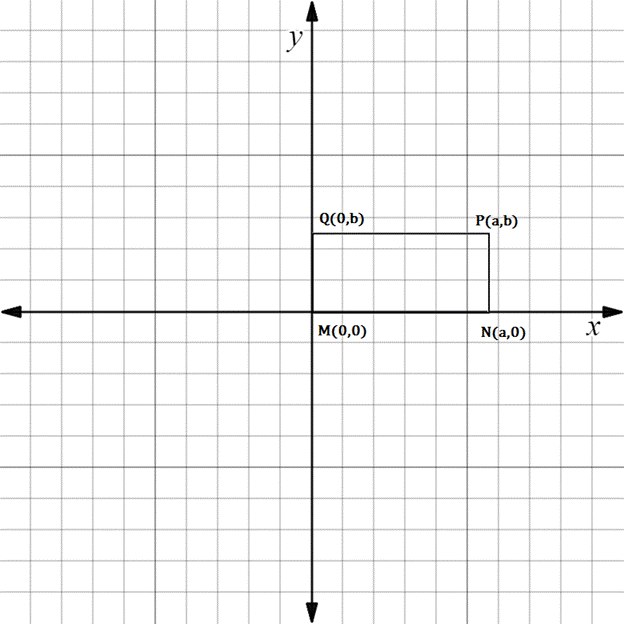Chapter 10.3, Problem 9EElementary Geometry For College St...

7th Edition
Alexander + 2 others
ISBN: 9781337614085

Solutions

Chapter
SectionElementary Geometry For College St...

7th Edition
Alexander + 2 others
ISBN: 9781337614085
Textbook Problem

In Exercises 5 to 10, the real numbers a, b, c, and d are positive.Consider the quadrilateral with vertices at M 0 ,   0 , N a ,   0 , P a ,   b , and Q 0 ,   b . Explain why M N P Q is a rectangle.To determine

To explain:

MNPQ is a rectangle.

Explanation

The given diagram is shown below.

To prove MNPQ is a rectangle, we have to prove the following statement.

Opposite sides are equal and parallel.

MN is parallel to QP.

That is slope of MQ is equal to slope of NP.

MQ is parallel to NP.

That is slope of MQ is equal to slope of NP.

Find the slope of MN.

x1,y1=M0, 0

x2,y2=Na, 0

Formula for slope joining the line segment.

Slope is denoted by m.

m=y2-y1x2-x1

Here x1x2

Substituting the x and y co-ordinates.

mMN=0-0a-0

mMN=0

Then, find the slope of QP.

x1,y1=Q0, b

x2,y2=Pa, b

Substituting the x and y co-ordinates.

mQP=b-ba-0

mQP=0a

mQP=0

mMN=mQP

Hence, MN and QP are parallel.

Find the slope of MQ.

x1,y1=M0, 0

x2,y2=Q0, b

Substituting the x and y co-ordinates in the slope formula.

mMQ=b-00-0

mMQ=not defined

Then, find the slope of NP.

x1,y1=Na, 0

x2,y2=Pa, b

Substituting the x and y co-ordinates.

mNP=b-0a-a

mNP=b0

mNP=undefined

mMQ=mNP

Hence, MQ and NP are parallel.

Now, we have to find the whether opposite sides are equal.

The distance between MN is equal to the distance between QP.

The distance between MQ is equal to the distance between NP.

Find the distance between M and N

Still sussing out bartleby?

Check out a sample textbook solution.

See a sample solution

The Solution to Your Study Problems

Bartleby provides explanations to thousands of textbook problems written by our experts, many with advanced degrees!

Get Started

Define the alpha level and the critical region for a hypothesis test.

Statistics for The Behavioral Sciences (MindTap Course List)

If a line is horizontal, then its slope is.

Mathematical Applications for the Management, Life, and Social Sciences

Evaluate the integral. 12(x22x)dx

Single Variable Calculus: Early Transcendentals